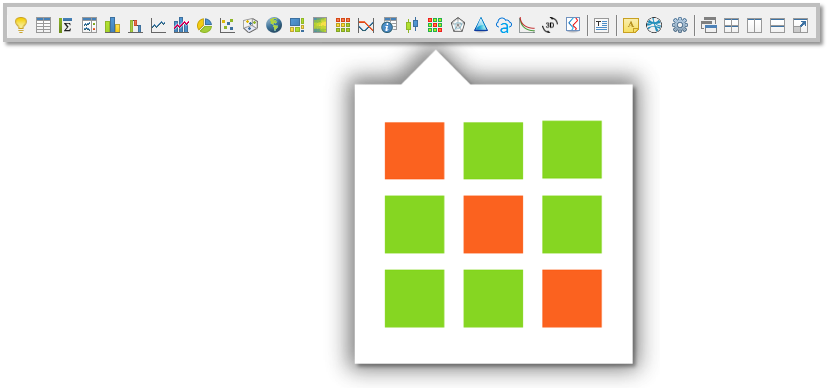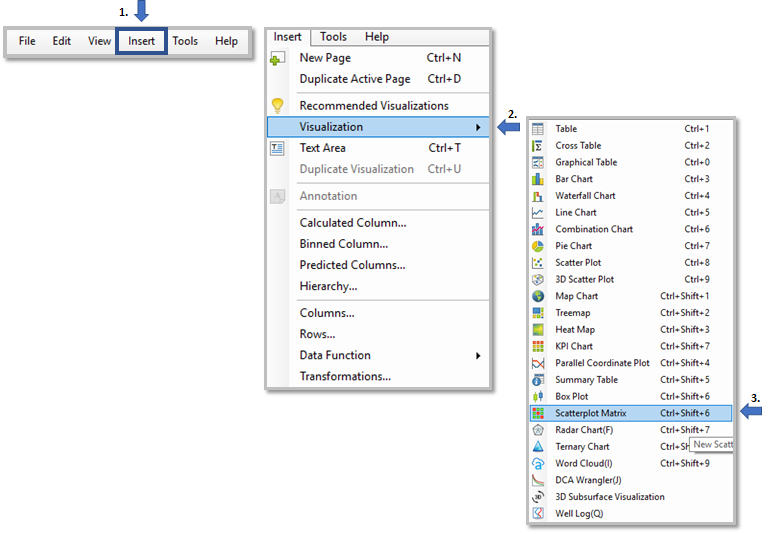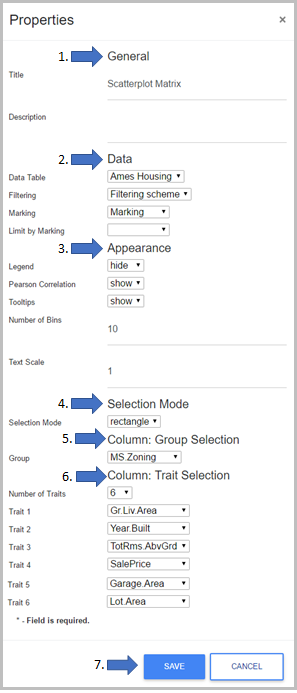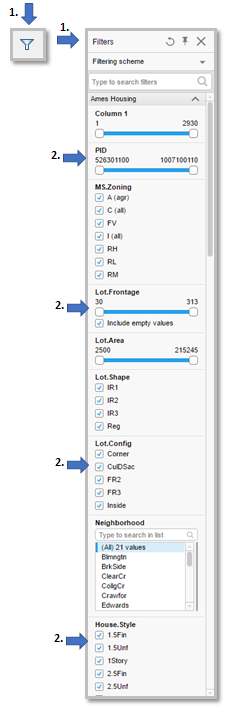Scatter Plot Matrices are a great way to roughly determine if you have a linear correlation between multiple variables. This is particularly helpful in pinpointing specific variables that might have a similar correlation. Data is divided into groups with each groups containing a specified number of traits. These traits are displayed in a matrix format so that traits can be compared. Along the diagonal, there are histograms for each trait. Above the cells of the histogram are the labels for the traits. Above the cells of the scatterplots is the Pearson Coefficient Score. Groups are colored differently.

### Purpose

Use the scatterplot matrix extension to understand the relationship between parameters. This visualization creates a scatter plot per each combination of variable allowing got identify correlation.

#### Steps to run:

1. Click the Scatterplot icon on the toolbar

####Another way to access Scatterplot Matrix Visualization

1. Click "Insert" on the top menu bar.
2. Go to "Visualization
3. Click Scatterplot Matrix.2. Click on the Properties menu on the top right corner of the Scatterplot Matrix visualization, and select each of the parameters below.#### Scatterplot properties Inputs

1. General
•  Title: the title of the visualization
•  Description: Add a description of this visualization
2. Data
• Data Table: Add the data table.
• Filtering, Marking, or limit by marking.
3. Appearance
• Show/Hide Legend
• Show/Hide Pearson Correlation
• Show/Hide Tooltips
• Number of Bin for the Histogram(Traits) - How many bars on the Histogram
• Text scale - Size of the text ( Pearson Correlation Score and Traits title)
4. Selection Mode
•  Choose either Pointer or Rectangle.
•  The Pointer selection mode Only selects the Histogram(traits) visualization.
•  The  Rectangle selection mode is able to select both scatterplots and HIstograms for further analysis.
5. Column: Group Selection
• Choose the column group
• This setting will specify what to use to distinguish between groups
6. Column: Trait Selection
• Number of traits: This is where you can add more traits to your visualization.
• Note when adding more or fewer traits click save for the visualizations to save the change, then go back to add the traits.
• Traits 1,2,3, etc. - These are the traits that are going to be shown in the visualization. (HIstogram, Diagonal)
7. Click Save after selecting all the settings.

#### Outputs:

• One Scatterplot Matrix Visualization
• Histogram (Diagonal) - Traits
• Scatterplot - Correlation between the multiple variables.
• (Above each Scatterplot is a Pearson Coefficient Score)

Example: This Image was taken from "Scatterplot Matrix Example" template from the Exchange.ai Portal. - Below is a link.#### How do I mark on the visualization?

To mark on the Scatterplot visualization, the settings in the properties menu under "Selection Mode" must be on the rectangle.

• This mode will draw a rectangle box when selecting on the scatterplot.
• To know if this mode is activated there will be a (+) when panning across scatterplot visualization.

To mark on the Histogramit could either be pointer or rectangle settings in the properties menu under Selection Mode.

• Pointer/Rectangle selects one bar on the histogram.

#### How to filter a subset of data

1. Open the filter panel by clicking the filter icon on the top bar.
2. Choose the correct filtering scheme.
• This will automatically filter the visualization upon selection.Link for template example on the Exchange.ai Portal below:

Scatter Plot Matrix Template Example

For additional information on RAI Scatter Plot Matrix documentation, click here